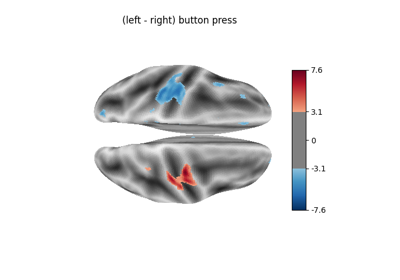Note

This page is a reference documentation. It only explains the class signature, and not how to use it. Please refer to the user guide for the big picture.

# nilearn.glm.Contrast#

class nilearn.glm.Contrast(effect, variance, dim=None, dof=10000000000.0, contrast_type='t', tiny=1e-50, dofmax=10000000000.0)[source]#

The contrast class handles the estimation of statistical contrasts on a given model: student (t) or Fisher (F).

The important feature is that it supports addition, thus opening the possibility of fixed-effects models.

The current implementation is meant to be simple, and could be enhanced in the future on the computational side (high-dimensional F contrasts may lead to memory breakage).

__init__(effect, variance, dim=None, dof=10000000000.0, contrast_type='t', tiny=1e-50, dofmax=10000000000.0)[source]#

Construct instance.

Parameters:
effectarray of shape (contrast_dim, n_voxels)

The effects related to the contrast.

variancearray of shape (n_voxels)

The associated variance estimate.

dimint or None, optional

The dimension of the contrast.

dofscalar, optional

The degrees of freedom of the residuals. Default=DEF_DOFMAX

contrast_type{‘t’, ‘F’}, optional

Specification of the contrast type. Default=’t’.

tinyfloat, optional

Small quantity used to avoid numerical underflows. Default=DEF_TINY

dofmaxscalar, optional

The maximum degrees of freedom of the residuals. Default=DEF_DOFMAX.

effect_size()[source]#

effect_variance()[source]#

stat(baseline=0.0)[source]#

Return the decision statistic associated with the test of the null hypothesis: (H0) ‘contrast equals baseline’.

Parameters:
baselinefloat, optional

Baseline value for the test statistic. Default=0.0.

Returns:
stat1-d array, shape=(n_voxels,)

statistical values, one per voxel.

p_value(baseline=0.0)[source]#

Return a parametric estimate of the p-value associated with the null hypothesis (H0): ‘contrast equals baseline’, using the survival function.

Parameters:
baselinefloat, optional

Baseline value for the test statistic. Default=0.0.

Returns:
p_values1-d array, shape=(n_voxels,)

p-values, one per voxel

one_minus_pvalue(baseline=0.0)[source]#

Return a parametric estimate of the 1 - p-value associated with the null hypothesis (H0): ‘contrast equals baseline’, using the cumulative distribution function, to ensure numerical stability.

Parameters:
baselinefloat, optional

Baseline value for the test statistic. Default=0.0.

Returns:
one_minus_pvalues1-d array, shape=(n_voxels,)

one_minus_pvalues, one per voxel

z_score(baseline=0.0)[source]#

Return a parametric estimation of the z-score associated with the null hypothesis: (H0) ‘contrast equals baseline’.

Parameters:
baselinefloat, optional,

Baseline value for the test statistic. Default=0.0.

Returns:
z_score1-d array, shape=(n_voxels,)

statistical values, one per voxel

## Examples using `nilearn.glm.Contrast`#Example of surface-based first-level analysis

Example of surface-based first-level analysisSurface-based dataset first and second level analysis of a dataset

Surface-based dataset first and second level analysis of a dataset